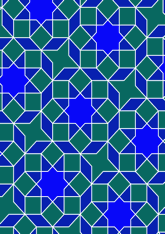# Afghanistan - the shrine at Mazar-e Sharifdata16/F86

## Geometry

• The symmetry group of the tiling is *442 (p4m).
• All the internal angles of the constituent polygons are a multiple of 45°.
• Contains two squares.
• Contains two regular two-pointed star polygons with vertex angle of 45°.
• Contains one regular four-pointed star polygon with vertex angle of 45°.
• Contains one regular 8-pointed star polygon with vertex angle of 90°.
• There is one non-regular reflective tile (including one kite).
• The tiling satisfies the two-colour condition.
• The tiling is edge-to-edge.
• As drawn, contains about 290 polygons.

## References

Publications referenced: